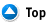Objective: IB - Primary Years Programme (PYP) PYP is for students aged 3 to 12. It provides schools with a curriculum framework of essential elements — the knowledge, concepts, skills, attitudes, and action that young students need to equip them for successful lives, both now and in the future. Schools work with the five elements to construct a rigorous and challenging primary curriculum for international education. IB - Middle Years Programme (MYP) MYP emphasizes intellectual challenge. It encourages students aged 11 to 16 to make practical connections between their studies and the real world, preparing them for success in further study and in life.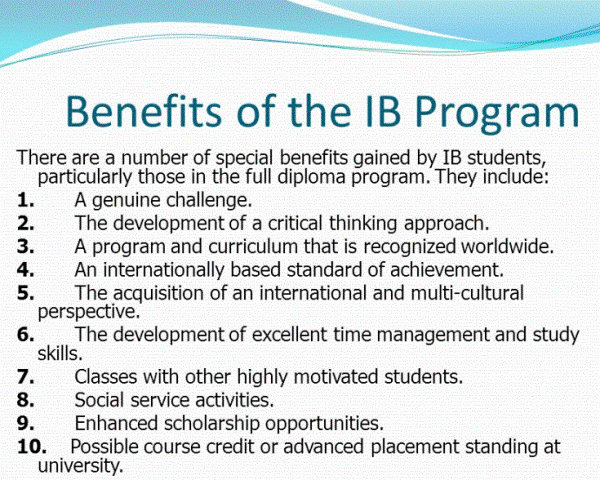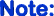If you are IB/IBT/AP student and your marks less than 80%, not worth to study in these Programme!

• IB - Diploma Programme (DP)

DP is an assessed programme for students aged 16 to 19. It is respected by leading universities across the globe.

What the DP offers students
Through the DP, schools are able to develop students who:

• have excellent breadth and depth of knowledge
• flourish physically, intellectually, emotionally and ethically
• explore the nature of knowledge through the programme’s unique theory of knowledge course.NallPro's Sample Worksheets! For IB & University MathWork Sheet 1PDF Printable versionWork Sheet 2PDF Printable versionWork Sheet 3PDF Printable version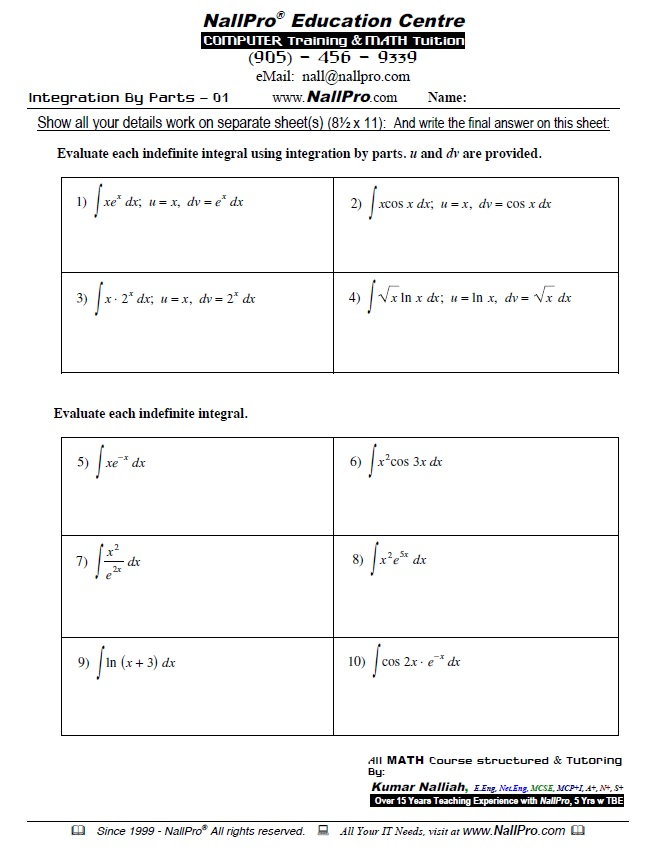Work Sheet 4PDF Printable version

IB - Diploma Programme (DP) You Will Learn...
 In Advanced Functions...(Course Breakdown) Functions: Characteristics and Properties Functions Exploring Absolute Value Properties of Graphs of Functions Sketching Graphs of Functions Inverse Relations Piecewise Functions Exploring Operations with Functions Functions: Understanding Rates of Change Determining Average Rate of Change Estimating Instantaneous Rates Using Rates of Change Solving Problems Involving Rates of Change Polynomial Functions Exploring Polynomial Functions Characteristics of Polynomial Functions Properties of Graphs of Functions Polynomial Functions in Factored Form Transformations of Cubic and Quartic Functions Dividing Polynomials Factoring Polynomials Factoring a Sum or Difference of Cubes Polynomial Equations and Inequalities Solving Polynomial Equations Solving Linear Inequalities Solving Polynomial Inequalities Rates of Change in Polynomial Functions Rational Functions, Equations, and Inequalities Graphs of Reciprocal Functions Exploring Quotients of Polynomial Functions Graphs of Rational Functions of the Form f(x) Solving Rational Equations Solving Rational Inequalities Rates of Change in Rational Functions Trigonometric Functions Radian Measure Radian Measure and Angles on the Cartesian Plane Exploring Graphs of the Primary Trigonometric Functions Transformations of Trigonometric Functions Exploring Graphs of the Reciprocal Trigonometric Functions Modelling with Trigonometric Functions Rates of Change in Trigonometric Functions Trigonometric Identities and Equations Exploring Equivalent Trigonometric Functions Compound Angle Formulas Double Angle Formulas Proving Trigonometric Identities Solving Linear Trigonometric Equations Solving Quadratic Trigonometric Equations Exponential and Logarithmic Functions Exploring the Logarithmic Function Transformations of Logarithmic Functions Evaluating Logarithms Laws of Logarithms Solving Logarithmic Equations Solving Problems with Exponential and Logarithmic Functions Rates of Change in Exponential and Logarithmic Functions In Data Management...(Course Breakdown) Tools for Data Management Statistics of One/Two Variable Permutations and Organized Counting Combinations and the Binomial Theorem Probability Distributions The Normal Distribution In Calculus...(Course Breakdown) Limits The Limit of a Function Properties of Limits Limit of continuity at a point One-Side Limits Limit as x Approaches to Infinity Differential calculus First Principles Rules of Power, Sum and Difference Product Rule and Quotient Rule Chain Rule Derivative of Trigonometric Functions Derivative of Exponential Functions Derivative of The Natural Log Functions Implicit Differentiation Higher Order Derivative Differential Calculus and Curve Sketching Tangents and Normals Curve Sketching - Increasing and Decreasing Functions Curve Sketching - Global Maxima and Minima The Second Derivative and its Application Rational Functions - Sketching the Graph Applications of Differential Calculus Rates Of Change Applied Rates of Change Kinematics - Motion Along a Straight Line Applied Maxima and Minima Problems Integration and Its Applications Antidifferentiation & The Indefinite Integral Determining The Indefinite Integral The Definite Integral Applications of Integration Introduction to the Area Beneath a Curve The Definite Integral and Areas Applications to Kinematics In Vectors...(Course Breakdown) An Introduction to Vectors Addition and Subtraction of Vectors Multiplication of a Vector by a Scalar Properties of Vectors Vectors in R2 and R3 Operations with Algebraic Vectors in R2 and R3 Linear Combinations and Spanning Sets Applications of Vectors Vectors as Forces Velocity The Dot Product of Two Geometric/Algebraic Vectors Scalar and Vector Projections The Cross Product of Two Vectors Applications of the Dot Product and Cross Product Equations of Lines and Planes Vector and Parametric Equations of a Line in R2 Cartesian Equation of a Line Vector, Parametric, and Symmetric Equations of a Line in R3 Vector and Parametric Equations of a Plane The Cartesian Equation of a Plane Sketching Planes in R3 Extension Concepts...(Course Breakdown) Complex Numbers Integration by Parts Integration Applications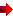Register ON-LINE